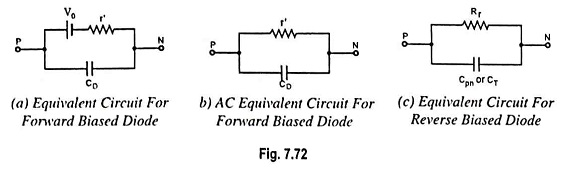## Equivalent Circuit of a Diode:

It is usually advantageous to replace a device or system by its equivalent circuit. An equivalent circuit of a device or a system is a combination of electric elements properly chosen to best represent the actual terminal characteristics of the device or the system. Once the equivalent circuits is determined, the device can be replaced by its Equivalent Circuit of a Diode without severely affecting the behavior of the overall system.We have seen that a forward-biased junction offers a dynamic or ac resistance r′ = (r + rB) and possesses diffusion capacitance CD which comes into picture only when frequency of the applied voltage is very high. Hence, it can be represented by the Equivalent Circuit of a Diode shown in Fig. 7.72 (a). An opposing battery has been connected in series with r’ to account for the junction harrier potential V0. The complete equivalent circuits for a forward biased diode is shown in Fig. 7.72(a).

The equivalent circuits for the forward-biased diode may be modified to form a small-signal ac equivalent circuits. This circuit is employed for diodes which are maintained in a forward-bias condition, but which are subjected to small variations in voltage V and current I. The small-signal ac Equivalent Circuit of a Diode is drawn [Fig. 7.72 (b)] by dropping the opposing battery representing the junction barrier potential V0 from the circuit shown in Fig. 7.72 (a).

A reverse-biased diode can be simply represented by the reverse resistance Rr in parallel with the depletion layer capacitance Cpn or CT. This is illustrated in Fig. 7.72 (c).

Scroll to Top